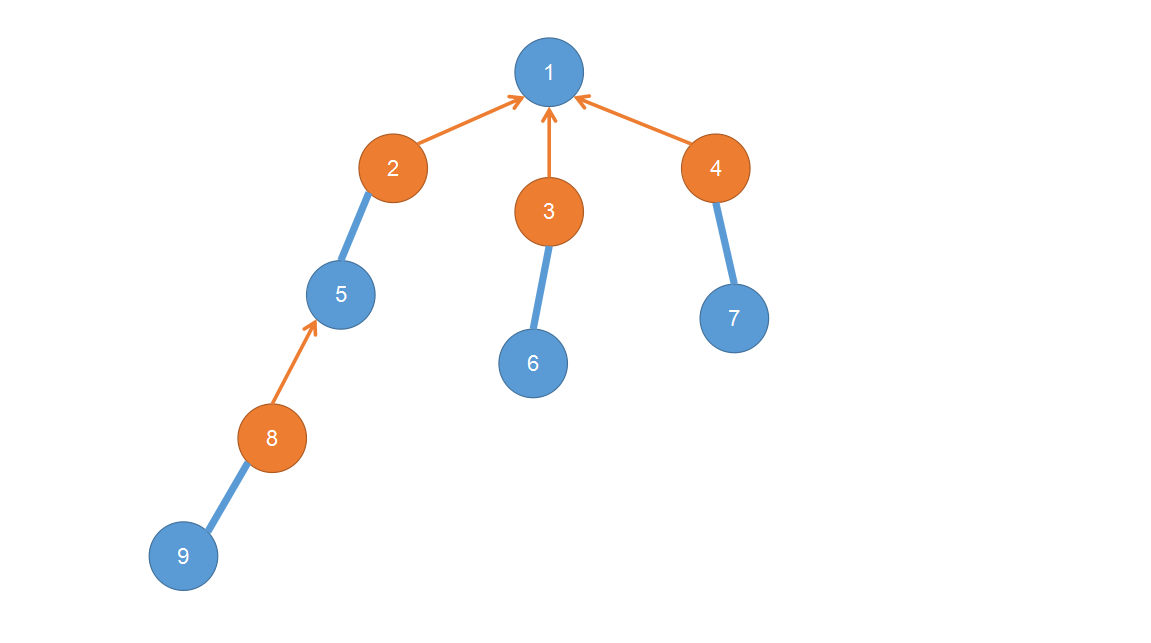# 算法流程：

• 从一个没有匹配出发，初始定义该点的颜色为黑。
for(int i=1;i<=n;++i) if(!match[i]&&solve(i)) ++ans;

• 每一次从队列里取出节点 $u$ 进行更新：
• 如果相邻点 $v$ 在当前轮还没有被染色

• 如果 $v$ 被匹配了

那么将匹配拆散，$v$ 当前为白点，将其匹配的点染成黑点，并扔进队列继续匹配，如果 $v$ 的匹配点找到了合适的匹配，那么 $v$ 可以和当前的起点 $i$ 匹配，将多增加一组匹配。

q.push(match[v]),col[match[v]]=1;

• 如果 $v$ 没有匹配

将 $v$ 匹配给起点 $i$ ，至于怎么维护可以每一个点记录 $pre$ ，然后暴力跳链更新：

for(int las;u;v=las,u=pre[v])
las=match[u],match[u]=v,match[v]=u;
return true;

• 如果相邻点 $v$ 的颜色是白

那么不用管，因为遇见了一个偶环。

• 如果相邻点 $v$ 的颜色是黑

• 如果 $v$ 和 $u$ 已经是同一个花里的了

那么不用管。

• 否则

暴力开花，先找到 $lca$ ，显然花将包括 $u$ 到 $v$ 路径上的所有点 (花)。

找到 $lca$ 后，分别从 $u$ 和 $v$ 开始往上面跳，并更新 $pre$ ，由于是花，花内的 $pre$ 一定是双向的，跳的时候还需要检查颜色，如果跳到的点 $x$ 是白色，那么染色为黑扔进队列，因为将花看做点的话是可以往外面匹配的，由于我们不确定是花中的哪个节点往外匹配，故均染色为黑。

注意跳的时候还需要更新并查集的 $fa$ 。# 一些题目

## $\rm{Code_1:}$

namespace _ {
queue <int> q;
int tim,fa[N],vis[N],pre[N],col[N],match[N];
inline int find(int x) {
while(x!=fa[x]) x=fa[x]=fa[fa[x]];
return x;
}
inline int lca(int x,int y) {
++tim,x=find(x),y=find(y);
for(;;swap(x,y)) {
if(!x) continue;
if(vis[x]==tim) return x;
vis[x]=tim,x=match[x]?find(pre[match[x]]):0;
}
}
inline void blossom(int x,int y,int t) {
while(find(x)!=t) {
pre[x]=y,y=match[x];
if(col[y]==2) col[y]=1,q.push(y);
fa[x]=fa[y]=t,x=pre[y];
}
}
inline bool solve(int s) {
memset(pre,0,sizeof(pre));
memset(col,0,sizeof(col));
for(int i=1;i<=n;++i) fa[i]=i;
while(!q.empty()) q.pop();
q.push(s),col[s]=1;
while(!q.empty()) {
int u=q.front();q.pop();
for(int v:G[u]) {
if(!col[v]) {
col[v]=2,pre[v]=u;
if(!match[v]) {
for(int las;u;v=las,u=pre[v])
las=match[u],match[u]=v,match[v]=u;
return true;
} else q.push(match[v]),col[match[v]]=1;
} else if(col[v]==1&&find(u)!=find(v)) {
int t=lca(u,v);
blossom(u,v,t),blossom(v,u,t);
}
}
}
return false;
}

} using namespace _;

int main() {
IN(n),IN(m);
for(int i=1;i<=n;++i) if(!match[i]&&solve(i)) ++ans;
printf("%d\n",ans);
return puts(""),0;xuyaozhuyi
}


## $\rm{Code_2:}$

namespace Flower_tree {
queue <int> q;
int tim,fa[N],vis[N],pre[N],col[N],match[N];
inline int find(int x) {
while(x!=fa[x]) x=fa[x]=fa[fa[x]];
return x;
}
inline int lca(int x,int y) {
++tim,x=find(x),y=find(y);
for(;;swap(x,y)) {
if(!x) continue;
if(vis[x]==tim) return x;
vis[x]=tim,x=match[x]?find(pre[match[x]]):0;
}
}
inline void blossom(int x,int y,int t) {
while(find(x)!=t) {
pre[x]=y,y=match[x];
if(col[y]==2) col[y]=1,q.push(y);
fa[x]=fa[y]=t,x=pre[y];
}
}
inline bool check(int s) {
memset(pre,0,sizeof(pre));
memset(col,0,sizeof(col));
for(int i=1;i<=L;++i) fa[i]=i;
while(!q.empty()) q.pop();
q.push(s),col[s]=1;
while(!q.empty()) {
int u=q.front();q.pop();
for(int v:G[u]) {
if(!col[v]) {
col[v]=2,pre[v]=u;
if(!match[v]) {
for(int las;u;v=las,u=pre[v])
las=match[u],match[u]=v,match[v]=u;
return true;
} else q.push(match[v]),col[match[v]]=1;
} else if(col[v]==1&&find(u)!=find(v)) {
int t=lca(u,v);
blossom(u,v,t),blossom(v,u,t);
}
}
}
return false;
}
inline int solve() {
int ans=0;
for(int i=1;i<=L;++i) if(!match[i]&&check(i)) ++ans;
return ans;
}
} using namespace Flower_tree;

inline void Main() {
memset(match,0,sizeof(match)),
memset(vis,0,sizeof(vis)),tim=0;
IN(n),IN(m),IN(e),L=n+m+m+m;
for(int i=1;i<=L;++i) G[i].clear();
int x,y;
for(int i=1;i<=e;++i) {
IN(x),IN(y);
}
for(int i=1;i<=m;++i)
printf("%d\n",solve()-n);
for(int i=1;i<=n;++i) printf("%d ",(match[i]-n-1)%m+1);
return puts(""),void();
}

int T;
int main() {
IN(T);
while(T--) Main();
return 0;
}


QAQ